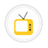you are here->home->Physical Sciences->Advanced Mechanics Virtual LabRigidity Modulus of The Suspension Wire of A Torsion Pendulum An experiment to find out the rigidity modulus of the suspension wire of a torsion pendulum in different environments.Young's Modulus-NonUniform BendingAim of this experiment is to find the Young's modulus of the given material by non-uniform bending using pin and microscope method.Compound Pendulum- SymmetricA rigid body with distributed mass able to freely pivot about a horizontal axis, which does not coincide with the center of gravity is called a compound pendulum. The compound pendulum is an interesting example of a pendulum that undergoes simple harmonicKater's pendulumIt is a compound pendulum constructed on the principle that centre of oscillation and centre of suspension are interchangable.The Kater's pendulum is used to measure the acceleration due to gravity more accurately.Young's Modulus-Uniform BendingAim of this experiment is to find the Young's modulus of the given material by uniform bending using pin and microscope method.Moment of inertia of a Torsion PendulumWhat is torsional oscillation? A body suspended by a thread or wire which twists first in one direction and then in the reverse direction, in the horizontal plane is called a torsional oscillation.Rigidity Modulus -Static Torsion Here Searle's static torsion apparatus is used to determine the rigidity modulus of the material of a given cylindrical rodCopyright @ 2019 Under the NME ICT initiative of MHRD